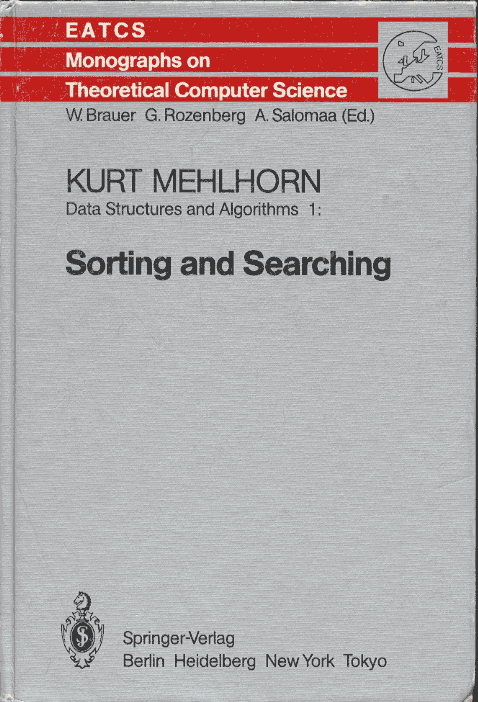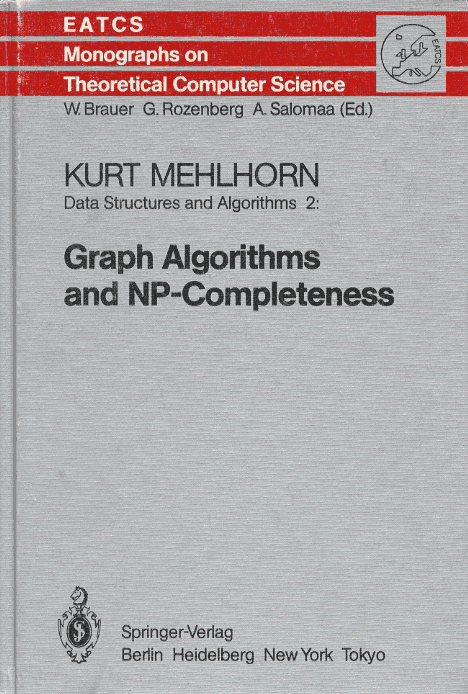Data Structures and Algorithms

# Data Structures and Algorithms Vol. I, II, and III

### Springer Verlag, EATCS Monographs

The books are out of print. The books were written on a type-writer. Some of my students converted the books to TeX. They added comments.### Vol. 1: Sorting and Searching

• Chapter 1, Foundations, ps, pdf
• Chapter 2, Sorting, ps, pdf
• Chapter 3, Sets, ps, pdf### Vol. 2: Graph Algorithms and NP-Completeness

• Chapter 4, Algorithms on Graphs, ps, pdf
• Chapter 5, Path Problems in Graphs and Matrix Multiplication, ps, pdf
• Chapter 6, NP-Completeness, ps, pdf### Vol. 3: Multi-dimensional Searching and Computational Geometry

• Chapter 7, Multidimensional Data Structures, ps, pdf
• Chapter 8, Computational Geometry, ps, pdf